# Combinatorics

Let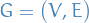be a bipartite graph with partitions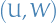.

Suppose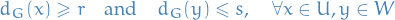where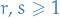.

Then, for non-empty $A ⊂ U,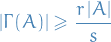Equality holds iff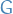is (r, s)-regular and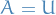. Let$ be the subgraph induced by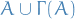, then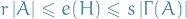where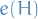is the number of edges in